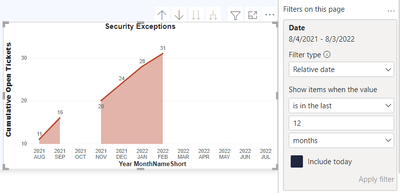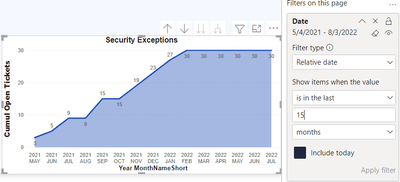cancel
Showing results for
Did you mean:Helper III

## Issue with cumulative measure and relative date filter

Hi everyone,

I have 2 measures that calculate the cumulative sum of the opened tickets. When I used these measures with a visual, the result isn't correct and I don't know how to solve it.

First measure

``````Cumulative Open Tickets =
VAR _lastDate = CALCULATE(MAX(DimDate[Date]),'Work Items')
RETURN
CALCULATE(
[Outstanding Tickets],
FILTER(
ALL(DimDate[Date]),
DimDate[Date] <= MAX(DimDate[Date])
),
DimDate[Date] <= _lastDate
)``````

Here, the result is always correct even when I apply a relative date filter but when there is no modification for a specific month, the measure returns nothing (not good)Here is the second measure

``````Cumul Open Tickets =
VAR _lastDate = MAX(DimDate[Date])
RETURN
CALCULATE(
[Outstanding Tickets],
FILTER(
ALLSELECTED(DimDate[Date]),
DimDate[Date] <= _lastDate
)
)``````

Here, the measure returns always a result, even when there is no modification (very good, that's what I want) but when I apply a filter with a relative date, the result changes because the measure restarts the calculation from the first virtual monthAs you see, the number of opened tickets is different according to the number of month. And that's not good because it doesn't reflect the reality. And this problem doesn't exist with the first measure.

What can I do to avoid the blank when there is no new event on the tickets and keep the real number of opened ticket (and not the number on the selected period)

Thanks

1 ACCEPTED SOLUTIONSuper User

I think the problem is the 'Work Items' filter being passed to MAX. Try

``````Cumulative Open Tickets =
VAR _lastDate = MAX ( DimDate[Date] )
RETURN
CALCULATE (
[Outstanding Tickets],
REMOVEFILTERS ( DimDate ),
DimDate[Date] <= _lastDate
)``````
6 REPLIES 6Super User

Try

``````Cumulative Open Tickets =
VAR _lastDate =
CALCULATE ( MAX ( DimDate[Date] ), 'Work Items' )
RETURN
CALCULATE (
[Outstanding Tickets],
REMOVEFILTERS ( DimDate ),
DimDate[Date] <= _lastDate
)``````Helper III

Hi @johnt75

No, with REMOVEFILTER the result is the sameSuper User

I think the problem is the 'Work Items' filter being passed to MAX. Try

``````Cumulative Open Tickets =
VAR _lastDate = MAX ( DimDate[Date] )
RETURN
CALCULATE (
[Outstanding Tickets],
REMOVEFILTERS ( DimDate ),
DimDate[Date] <= _lastDate
)``````Helper III

Very good @johnt75

Your DAX formula return the cumulative sum even if I apply a relative date 👍👏

But that blow my mind .....

Why

``MAX ( DimDate[Date] ) ``

and

``CALCULATE ( MAX ( DimDate[Date] ), 'Work Items' )``

has an impact on the final result?

If you can help me to understandSuper User

When using the Work Items table as a filter, only dates which appear in that table will be added to the filter context. So any dates which don't appear in Work Items will effectively be discarded from the Date table before running the MAX calculation. Combining this with having the months on the x-axis means that for a month where no dates exist in Work Items MAX(Date[Date]) will return blank, and so the rest of the calculation will also return blank.Helper III

Ok, very clear. Thanks for the explanationsAnnouncements#### Exclusive opportunity for Women!

Join us for a free, hands-on Microsoft workshop led by women trainers for women where you will learn how to build a Dashboard in a Day!#### Power Platform Conference-Power BI and Fabric Sessions

Join us Oct 1 - 6 in Las Vegas for the Microsoft Power Platform Conference.Top Solution Authors
Top Kudoed Authors
Users online (2,975)